# Faraday’s Law of Electromagnetic Induction

• Last Updated : 25 Feb, 2022

Magnetic flux through any surface placed in a magnetic field is defined as the number of magnetic lines of force crossing the surface normally. It is denoted by ‘ⲫ’ and its unit is weber (Wb). Electromagnetic induction is the phenomenon of production of induced emf due to a change of magnetic flux (number of magnetic field lines) connected to a closed circuit is called electromagnetic induction.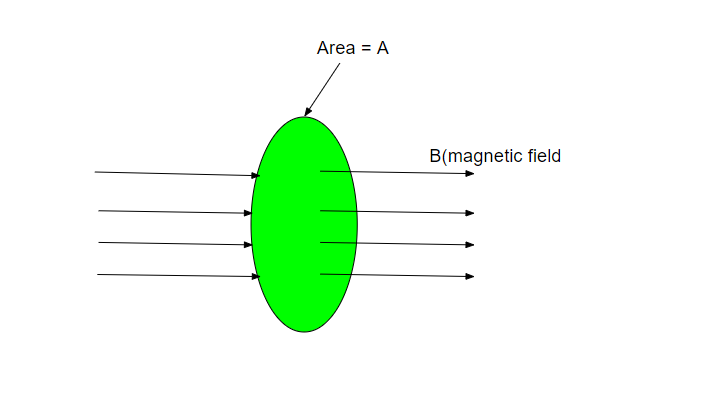Experiment 1: For doing this experiment Faraday took a circular coil and connected it with a galvanometer and a strong bar magnet. When the north pole of the bar magnet is moved towards the coil, the galvanometer showed deflection to the right side of the zero mark in the galvanometer. When the magnet is moved away from the coil again it showed deflection but in the opposite direction. Similarly, the experiment is done with the south pole of the bar magnet, again the deflection is observed but opposite to the direction shown by the north pole of the bar magnet. When the magnet is held stationary near the coil, no deflection is observed in the galvanometer.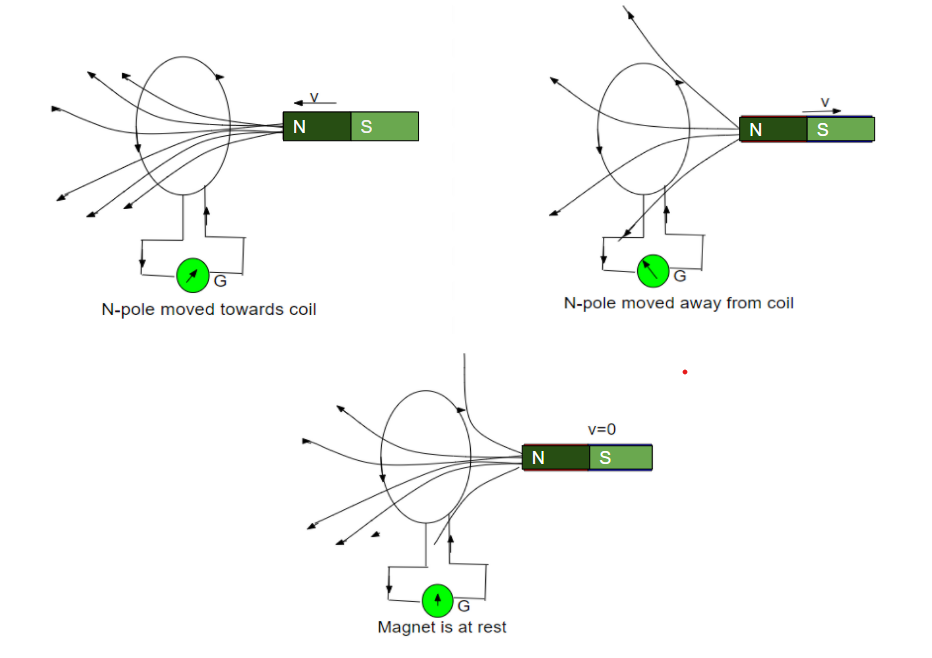Conclusion: As the magnet is moved closer to the coil the magnetic flux increases hence, an induced current setup in the coil in one direction. When the magnet is moved away from the coil, the magnetic flux decreases, hence an induced current set up in the coil in opposite direction. When the magnet is held stationary inside the coil, magnetic flux stops changing, hence induced current the coil becomes zero.

Experiment 2: In this experiment bar magnet is kept stationary and the coil is moved. The same result is observed as in experiment 1. When the relative motion between the magnet and coil is fast, the deflection in the galvanometer is larger and vice versa.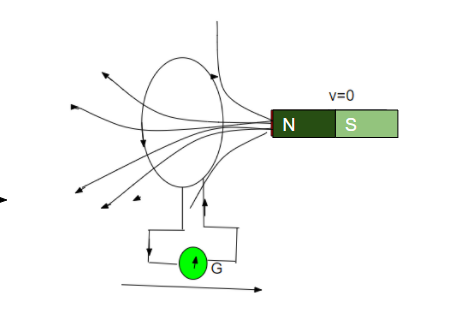Experiment 3: As you can see from the figure below. Two coils primary (p) and secondary (s), are wound on cylindrical support. The primary coil is connected with a key, a rheostat, and a battery. The secondary is connected with a galvanometer. When the key is pressed in the primary coil the galvanometer shows deflection in one direction. When the key is released, it again shows deflection but in the opposite direction. When the key is kept pressed steady current flows through the primary coils, the galvanometer does not show any deflection. When the current in the primary coil is increased with the help of rheostat, the induced current flows in the secondary coil in the same direction as that of the primary coil.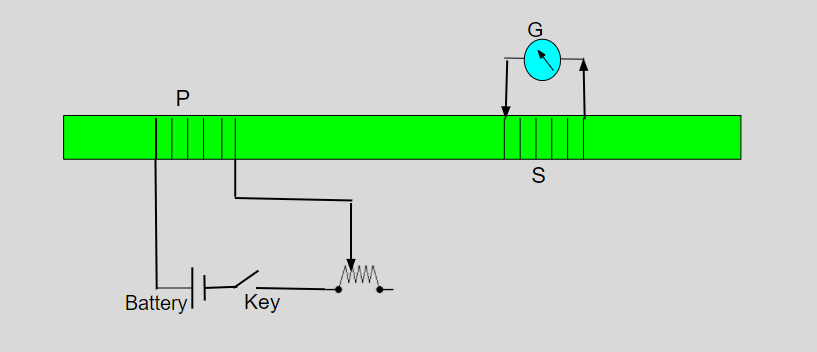### Faraday’s laws of electromagnetic induction

First law: It states that whenever the magnetic flux is linked with a closed-circuit change, an emf is induced in it which lasts only as long as the change in flux is taking place. If the circuit is closed then current also gets induced inside the circuit which is called ” Induced current”.

Magnetic fields can be changed by:

• Moving a bar magnet towards or away from the coil.
• Moving the coil into the magnetic field or outside the magnetic field.
• Rotating the coil relative to the magnet.
• Changing the area of a coil placed in the magnetic field.

Second law: It states that the magnitude of the induced emf is equal to the rate of change of magnetic flux linked with the closed circuit.

|∈| = dⲫ/dt

E = -N dⲫ/dt

E = -N (ⲫ2-ⲫ1)/t ⇢ (” t “is time)

Lenz’s law

Lenz states that the direction of induced current in a circuit is such that it opposes the change in magnetic flux. Lenz’s is named after the German physicist “Emil Lenz “, who formulated it in 1834. It is a scientific law that specifies the direction of induced current but states nothing about its magnitude.

E = -N(d∅/dt) ⇢ (negative sign indicates that the direction of induced emf is such that it opposes the change in magnetic flux)

• Induction motors
• Transformers
• Electric generators
• Hall effect meters
• Current clamps
• Induction cooking
• Induction welding
• Induction sealing
• Electric guitar and violin.

### Sample Problems

Question 1: The magnetic flux linked with a coil is changed from 2Wb to 0.2Wb in 0.5 seconds. Calculate the induced emf.

Δⲫ = 0.2-2 = 1.8wb

Δt = 0.5 sec

E = -(Δⲫ/Δt)

E= -1.8/0.5 volts

E= -3.6 volts

Therefore, induced emf will be -3.6 volts.

Question 2: In a coil of resistance 200, a current is induced by changing the magnetic flux through it as shown in the figure. Calculate the magnitude of change in flux through the coil.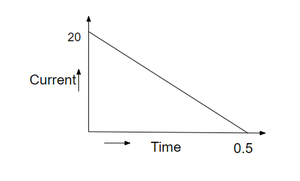Solution:

dq = – (N/R) dt

i= (1/R). (dq/dt)

Δⲫ = R.Δq

Δⲫ = 200 × (Area of circular graph)

Δⲫ = 200 × (1/2×20×0.5)

Δⲫ = 200 × 5

Δⲫ = 1000 Wb

Therefore, magnitude of change in flux is 1000 Wb.

Question 3: Calculate the emf induced in the wire. When a small piece of metal wire dragged across the gap between the pole pieces of a magnet in 0.6sec. The magnetic flux between the poles pieces is known to be 9×10-4 Wb.

Solution:

dt=0.5 s

dⲫ = 9×10-4-0 = 9×10-4 Wb

E = (dⲫ)/dt

E= (9×10-4)/0.6

E= 0.0036 V

Therefore, the induced emf 0.0036V

Question 4: A cylindrical bar magnet is kept along the axis of the circular coil. Give the reason, Why the current will not induce in the coil if the magnet is rotated about its axis.

Solution:

Current will not induce, because the magnetic flux linked with the circular coil does not change when magnet is rotated.

Question 5: Why the induced emf is also called back emf?

Solution:

Induced emf is also called back emf because induced emf always opposes any change in applied emf.

Question 6: Give the direction in which the induced current flows in the wire loop when the magnet moves towards it as shown in the figure.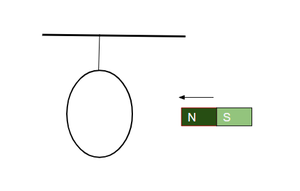Solution:

When seen from the magnet side, the induced current flows anticlockwise in the loop.

Question 7: Why are inductance coils made of copper?

Solution:

Copper has small resistance, so induced current set up will be large.

My Personal Notes arrow_drop_up